One of the most famous scientific discoveries was Newton's laws of motion. The laws allowed people to make predictions. For example, the acceleration for an object can be predicted given the applied force and the mass of the object. Making predictions remains a popular endeavour. This post explains the simplest way to predict an outcome for a new value, given a set of points.

To explain the concepts, data on apples and pears is generated. Underlying relations for the generated data are known. The known relations can be compared to the results from the regression.

## Generating data

The goal is to predict whether a fruit is an apple or a pear. Say we have a sample of size $n$. Say the sample consist of two properties for each fruit, namely

• height, and

• width.

The properties for the fruit at index $i$ are respectively denoted by $h_i$ and $w_i$. Prediction will be done for the fruit type, which is denoted by $y_i$. The sample indices can be visualised as follows.

IHWY
1$h_1$$w_1$$y_1$
2$h_2$$w_2$$y_2$
............
$n$$h_n$$w_n$$y_n Let half of the elements be apples and half of the elements be pears. So, n is even. Let$$y_{i} = \begin{cases} \text{apple} & \text{if$i$is odd}, \: \text{and} \\ \text{pear} & \text{if$i$is even}. \end{cases}$$Let the height and fruit type be correlated. To this end define$$h_{i} = \begin{cases} \text{one sample of size 1 drawn from } N(10, 1) & \text{if$Y_i$is apple}, \: \text{and} \\ \text{one sample of size 1 drawn from } N(12, 1) & \text{if$Y_iis pear}. \end{cases}$$Let the height and width also be correlated. Define the width to be 0.6 times the height. Specifically,$$w_i = 0.6 h_i.$$In Julia, this can be defined as begin using AlgebraOfGraphics using CairoMakie using DataFrames using Distributions: Binomial, Normal using GLM: LogitLink, @formula, coef, glm, lm, predict using Random: seed! using Statistics end r_2(x) = round(x; digits=2) r_2 (generic function with 1 method) df = let seed!(18) n = 12 I = 1:n Y = [i % 2 != 0 for i in I] H = r_2.([y == "apple" ? rand(Normal(10, 1)) : rand(Normal(12, 1)) for y in Y]) W = r_2.([0.6h for h in H]) DataFrame(; I, H, W, Y) end I H W Y 1 1 11.77 7.06 true 2 2 10.56 6.34 false 3 3 13.48 8.09 true 4 4 11.32 6.79 false 5 5 12.03 7.22 true 6 6 11.87 7.12 false 7 7 13.5 8.1 true 8 8 11.83 7.1 false 9 9 11.42 6.85 true 10 10 11.98 7.19 false 11 11 11.38 6.83 true 12 12 12.23 7.34 false ## Simple linear regression A simple linear regression fits a line through points in two dimensions. It should be able to infer the relation between H and W.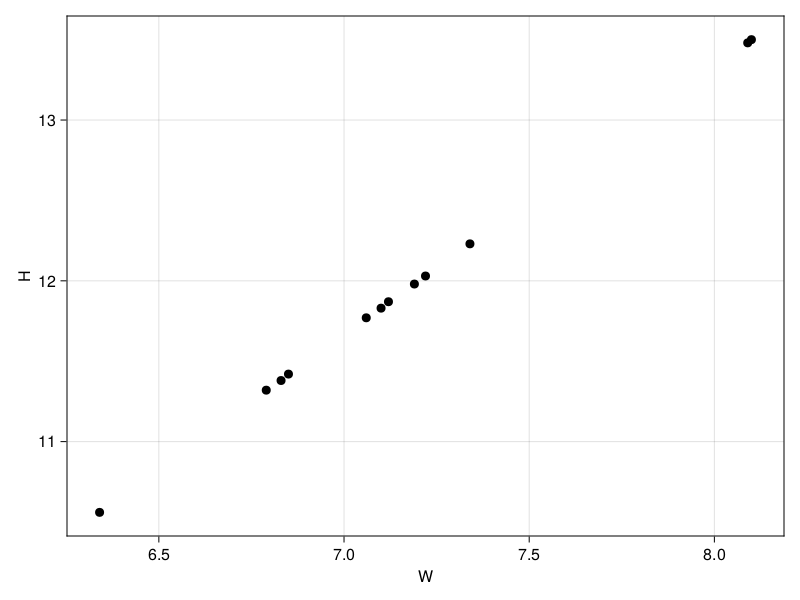The algorithmic way to fit a line is via the method of least squares. Any straight line can be described by a linear equation of the form y = p_1 x + p_0, where the first parameter p_0 is the intercept with y and the second parameter p_1 is the slope. Adding an error e, and rewriting gives$$\begin{aligned} y_i & = p_0 + p_1 x_i + e_i \\ e_i & = y_i - p_0 - p_1 x_i. \\ \end{aligned}$$An algorithm could now be defined to naively minimize the sum of all the errors$$S'(p_0, p_1) = \sum_{i=1}^n e_i,$$with respect to the choice of p_0 and p_1. This would not always result in a well fitted line because errors might cancel each other out. For example, when e_1 = 10 and e_2 = -10, then e_1 + e_2 = 0. This is solved by squaring the errors. The method of least squares minimizes$$S_l(p_0, p_1) = \sum_{i=1}^n e_i^2 = \sum_{i=1}^n (y_i - p_0 - p_1 x_i)^2$$with respect to the choice of p_0 and p_1 (Rice, 2006). The simplest estimator for the points is the mean. We can plot this and show horizontal lines for the errors.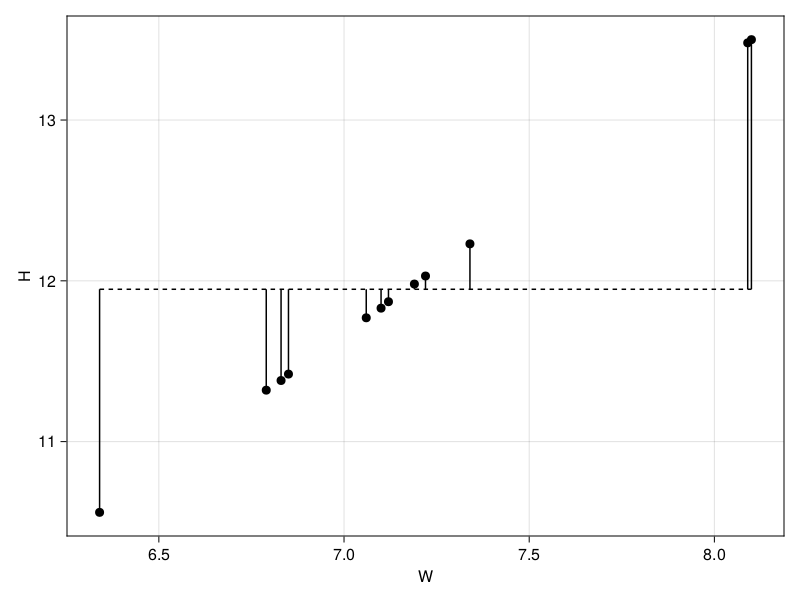We can generalize the sum of squares error calculation to$$S(U, V) = \sum_{i=1}^n (u_i - v_i)^2,$$for arrays U and V. S(U, V) = sum((U .- V).^2) S (generic function with 1 method) Then, the squared sum of the errors for this simplest estimator is r_2(S(df.H, repeat([mean(df.H)], length(df.H)))) 7.82 This error cannot be compared to other errors, since it is not standardized. A standardized metric would be the Pearson correlation coefficient r. See the blog post on correlations for more information. m1 = lm(@formula(H ~ W), df);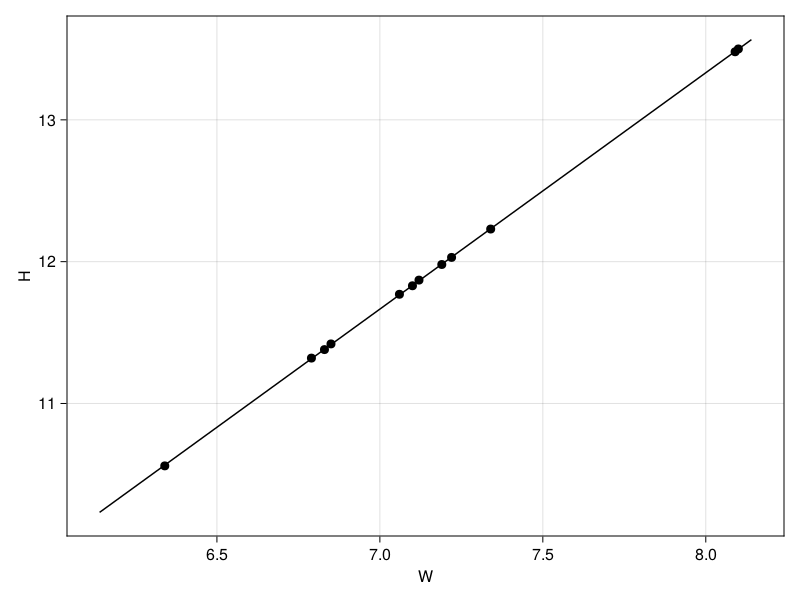""" predict_value(model, x) This is just a convenience function around GLM.predict. """ function predict_value(model, x) return first(skipmissing(predict(model, DataFrame(W = [x])))) end; The intercept and slope for the fitted line are intercept(linear_model) = coef(linear_model) intercept (generic function with 1 method) slope(linear_model) = coef(linear_model) slope (generic function with 1 method) r_2(intercept(m1)) -0.0 r_2(slope(m1)) 1.67 ## Binary logistic regression Next, lets try to estimate the relation between Y and W. The method of least squares is unable to calculate an error for "apple" and "pear". A workaround is to encode "apple" as 0 and "pear" as 1. A line can now be fitted. df_digits = let digits = [i % 2 != 0 ? 0 : 1 for i in df.I] df_digits = DataFrame(df) df_digits[!, :Y_digit] = digits df_digits end; m2 = lm(@formula(Y_digit ~ W), df_digits);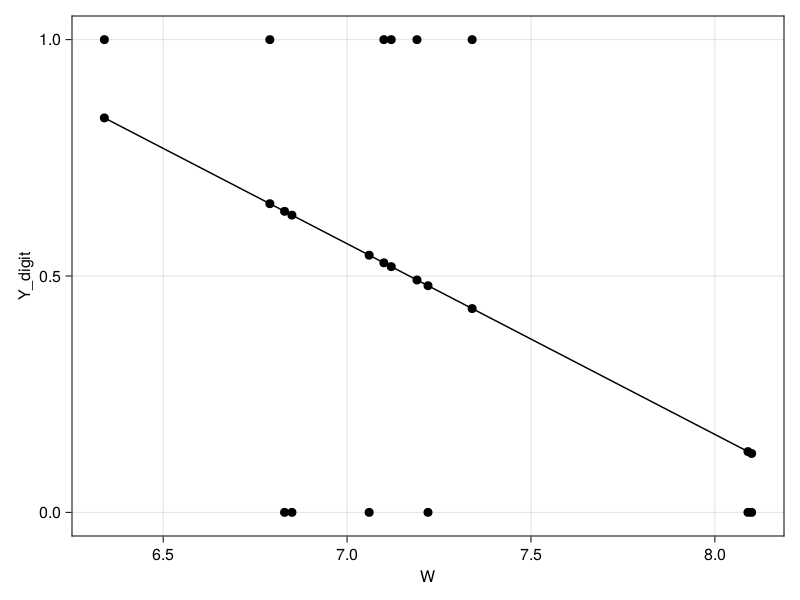As can be observed, the model does not take into account that Y is a binary variable. The model even predicts values outside the expected range, that is, values outside the range 0 to 1. A better fit is the logistic function. m3 = glm(@formula(Y_digit ~ W), df_digits, Binomial(), LogitLink());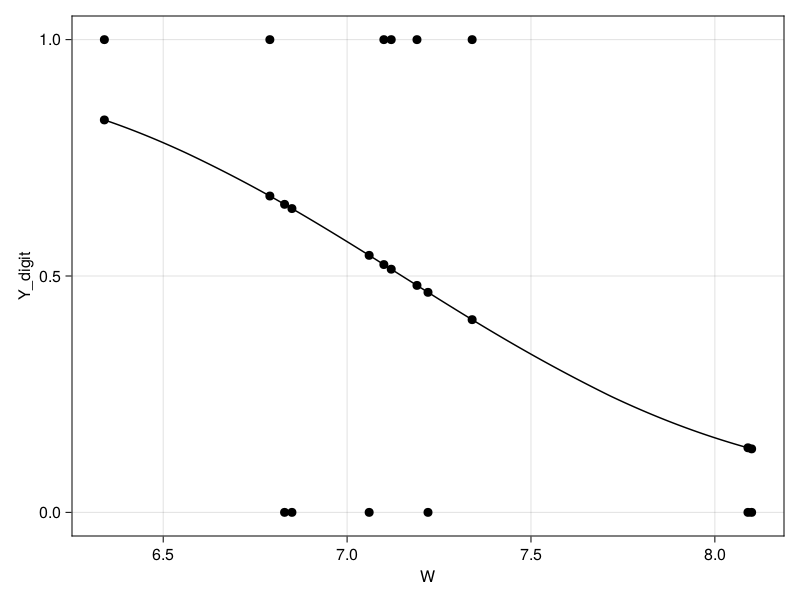The correlation coefficient r should not be used to compare the models, since the logistic model only predicts the class. In other words, the logistic model is a classifier. Classification accuracy is a better metric:$$\text{accuracy} = \frac{\text{number of correct predictions}}{\text{total number of predictions}} .$$accuracy(trues, pred) = count(trues .== pred) / length(pred) accuracy (generic function with 1 method) The threshold is set to 0.5 to get a binary prediction from both models. More precisely: let \text{pred}(x_i) denote the prediction for y_i, and y_i' denote the binary prediction for y_i. For each prediction$$y_i' = \begin{cases} 1 & \text{if0.5 < \text{pred}(x_i)$}, \: \text{and} \\ 0 & \text{if$\text{pred}(x_i) \leq 0.5$}. \\ \end{cases}$\$

binary_values(model) = [0.5 < x ? 1 : 0 for x in predict(model)]
binary_values (generic function with 1 method)
let
model = ["Linear regression", "Logistic regression"]
digits = df_digits.Y_digit
error = r_2.([S(digits, predict(m2)), S(digits, predict(m3))])
accuracy = r_2.([accuracy(digits, binary_values(m2)), accuracy(digits, binary_values(m3))])

DataFrame(; model, error, accuracy)
end
model error accuracy
1 "Linear regression" 2.54 0.58
2 "Logistic regression" 2.61 0.58

As can be seen, the error is lower for the logistic regression. However, for the accuracy both models score the same in this case. This is due to the fact that there is only a very small area where the linear and logistic model make different predictions. When this area becomes bigger (for example, when doing regression in multiple dimensions) or when more points lie in this area, then the accuracy for the logistic regression will be better compared to the linear regression.

## References

Rice, J. A. (2006). Mathematical statistics and data analysis. Cengage Learning.

Built with Julia 1.8.1 and

AlgebraOfGraphics 0.6.11
CairoMakie 0.8.13
DataFrames 1.3.4
Distributions 0.25.67
GLM 1.8.0